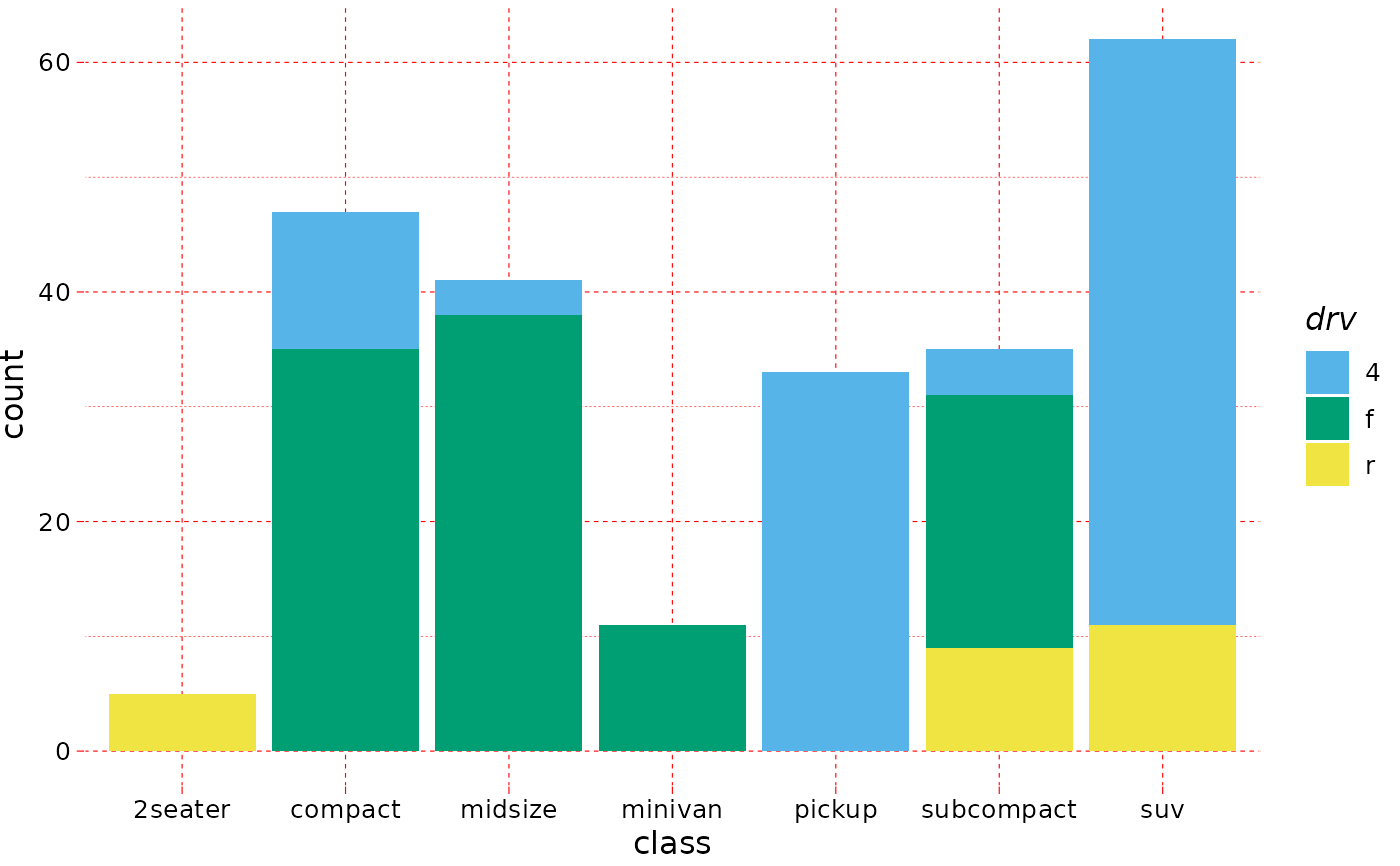The pander ships with a default theme when the 'unify plots' option is enabled via panderOptions, which is now also available outside of pander internals, like evals, eval.msgs or Pandoc.brew.

theme_pander(
base_size = 12,
base_family = "sans",
nomargin = TRUE,
ff = NULL,
fc = "black",
fs = NULL,
gM = TRUE,
gm = TRUE,
gc = "grey",
gl = "dashed",
boxes = FALSE,
bc = "white",
pc = "transparent",
lp = "right",
axis = 1
)

## Arguments

base_size base font size, given in pts. base font family suppress the white space around the plot (boolean) font family, like sans. Deprecated: use base_family instead. font color (name or hexa code) font size (integer). Deprecated: use base_size instead. major grid (boolean) minor grid (boolean) grid color (name or hexa code) grid line type (lty) to render a border around the plot or not background color (name or hexa code) panel background color (name or hexa code) legend position axis angle as defined in par(les)

## Examples

require("ggplot2")
if (require("pander")) {

p <- ggplot(mtcars, aes(x = mpg, y = wt)) +
geom_point()
p + theme_pander()

panderOptions("graph.grid.color", "red")
p + theme_pander()

p <- ggplot(mtcars, aes(wt, mpg, colour = factor(cyl))) +
geom_point()
p + theme_pander() + scale_color_pander()

ggplot(mpg, aes(x = class, fill = drv)) +
geom_bar() +
scale_fill_pander() +
theme_pander()
}
#> Loading required package: pander